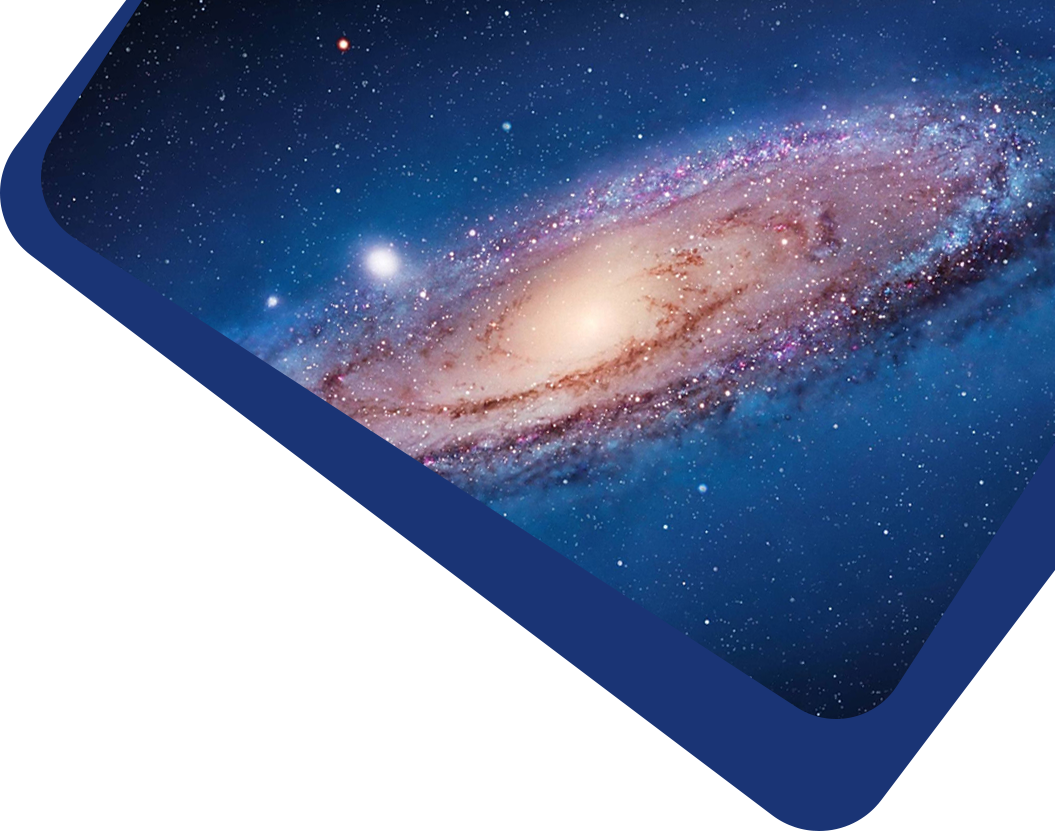Vol 22, No 12

## The Analytical Equation of the Three-point Correlation Function of Galaxies: to the Third Order of Density Perturbation

#### Shu-Guang Wu and Yang Zhang

Abstract

Applying functional differentiation to the density field with Newtonian gravity, we obtain the static, nonlinear equation of the three-point correlation function ζ of galaxies to the third order density perturbations. We make the equation closed and perform renormalization of the mass and the Jeans wavenumber. Using the boundary condition inferred from observations, we obtain the third order solution ζ(r, u, θ) at fixed u = 2, which is positive, exhibits a U-shape along the angle θ, and decreases monotonously along the radial r up to the range r ≤ 30 h−1 Mpc in our computation. The corresponding reduced Q(r, u, θ) deviates from 1 of the Gaussian case, has a deeper U-shape along θ, and varies non-monotonously along r. The third order solution agrees with the SDSS data of galaxies, quite close to the previous second order solution, especially at large scales. This indicates that the equations of correlation functions with increasing orders of density perturbation provide a stable description of the nonlinear galaxy system.

Keywords

(cosmology:) large-scale structure of universe – hydrodynamics – gravitation

Full Text
Refbacks